Set the objective of a conservation planning problem() to maximize the phylogenetic endemism of the features represented in the solution subject to a budget. This objective is similar to add_max_phylo_end_objective() except that emphasis is placed on representing species with geographically restricted evolutionary histories, instead representing as much evolutionary history as possible. This function was inspired by Faith (1992), Rodrigues et al. (2002), and Rosauer et al. (2009).

add_max_phylo_end_objective(x, budget, tree)

## Arguments

x

problem() (i.e., ConservationProblem) object.

budget

numeric value specifying the maximum expenditure of the prioritization. For problems with multiple zones, the argument to budget can be (i) a single numeric value to specify a single budget for the entire solution or (ii) a numeric vector to specify a separate budget for each management zone.

tree

phylo() object specifying a phylogenetic tree for the conservation features.

## Value

Object (i.e., ConservationProblem) with the objective added to it.

## Details

The maximum phylogenetic endemism objective finds the set of planning units that meets representation targets for a phylogenetic tree while staying within a fixed budget. If multiple solutions can meet all targets while staying within budget, the cheapest solution is chosen. Note that this objective is similar to the maximum features objective (add_max_features_objective()) in that it allows for both a budget and targets to be set for each feature. However, unlike the maximum feature objective, the aim of this objective is to maximize the total phylogenetic endemism of the targets met in the solution, so if multiple targets are provided for a single feature, the problem will only need to meet a single target for that feature for the phylogenetic benefit for that feature to be counted when calculating the phylogenetic endemism of the solution. In other words, for multi-zone problems, this objective does not aim to maximize the phylogenetic endemism in each zone, but rather this objective aims to maximize the phylogenetic endemism of targets that can be met through allocating planning units to any of the different zones in a problem. This can be useful for problems where targets pertain to the total amount held for each feature across multiple zones. For example, each feature might have a non-zero amount of suitable habitat in each planning unit when the planning units are assigned to a (i) not restored, (ii) partially restored, or (iii) completely restored management zone. Here each target corresponds to a single feature and can be met through the total amount of habitat in planning units present to the three zones.

## Mathematical formulation

This objective can be expressed mathematically for a set of planning units ($$I$$ indexed by $$i$$) and a set of features ($$J$$ indexed by $$j$$) as:

$$\mathit{Maximize} \space \sum_{i = 1}^{I} -s \space c_i \space x_i + \sum_{j = 1}^{J} m_b l_b \frac{1}{a_b} \\ \mathit{subject \space to} \\ \sum_{i = 1}^{I} x_i r_{ij} \geq y_j t_j \forall j \in J \\ m_b \leq y_j \forall j \in T(b) \\ \sum_{i = 1}^{I} x_i c_i \leq B$$

Here, $$x_i$$ is the decisions variable (e.g., specifying whether planning unit $$i$$ has been selected (1) or not (0)), $$r_{ij}$$ is the amount of feature $$j$$ in planning unit $$i$$, $$t_j$$ is the representation target for feature $$j$$, $$y_j$$ indicates if the solution has meet the target $$t_j$$ for feature $$j$$. Additionally, $$T$$ represents a phylogenetic tree containing features $$j$$ and has the branches $$b$$ associated within lengths $$l_b$$. Each branch $$b \in B$$ is associated with a total amount $$a_b$$ indicating the total geographic extent or amount of habitat. The $$a_b$$ variable for a given branch is calculated by summing the $$r_{ij}$$ data for all features $$j \in J$$ that are associated with the branch. The binary variable $$m_b$$ denotes if at least one feature associated with the branch $$b$$ has met its representation as indicated by $$y_j$$. For brevity, we denote the features $$j$$ associated with branch $$b$$ using $$T(b)$$. Finally, $$B$$ is the budget allocated for the solution, $$c_i$$ is the cost of planning unit $$i$$, and $$s$$ is a scaling factor used to shrink the costs so that the problem will return a cheapest solution when there are multiple solutions that represent the same amount of all features within the budget.

See objectives for an overview of all functions for adding objectives. Also, see targets for an overview of all functions for adding targets, and add_feature_weights() to specify weights for different features.

Other objectives: add_max_cover_objective(), add_max_features_objective(), add_max_phylo_div_objective(), add_max_utility_objective(), add_min_largest_shortfall_objective(), add_min_set_objective(), add_min_shortfall_objective()

## Examples

# load ape package
require(ape)

data(sim_pu_raster, sim_features, sim_phylogeny, sim_pu_zones_stack,
sim_features_zones)

# plot the simulated phylogeny
# \dontrun{
par(mfrow = c(1, 1))
plot(sim_phylogeny, main = "phylogeny")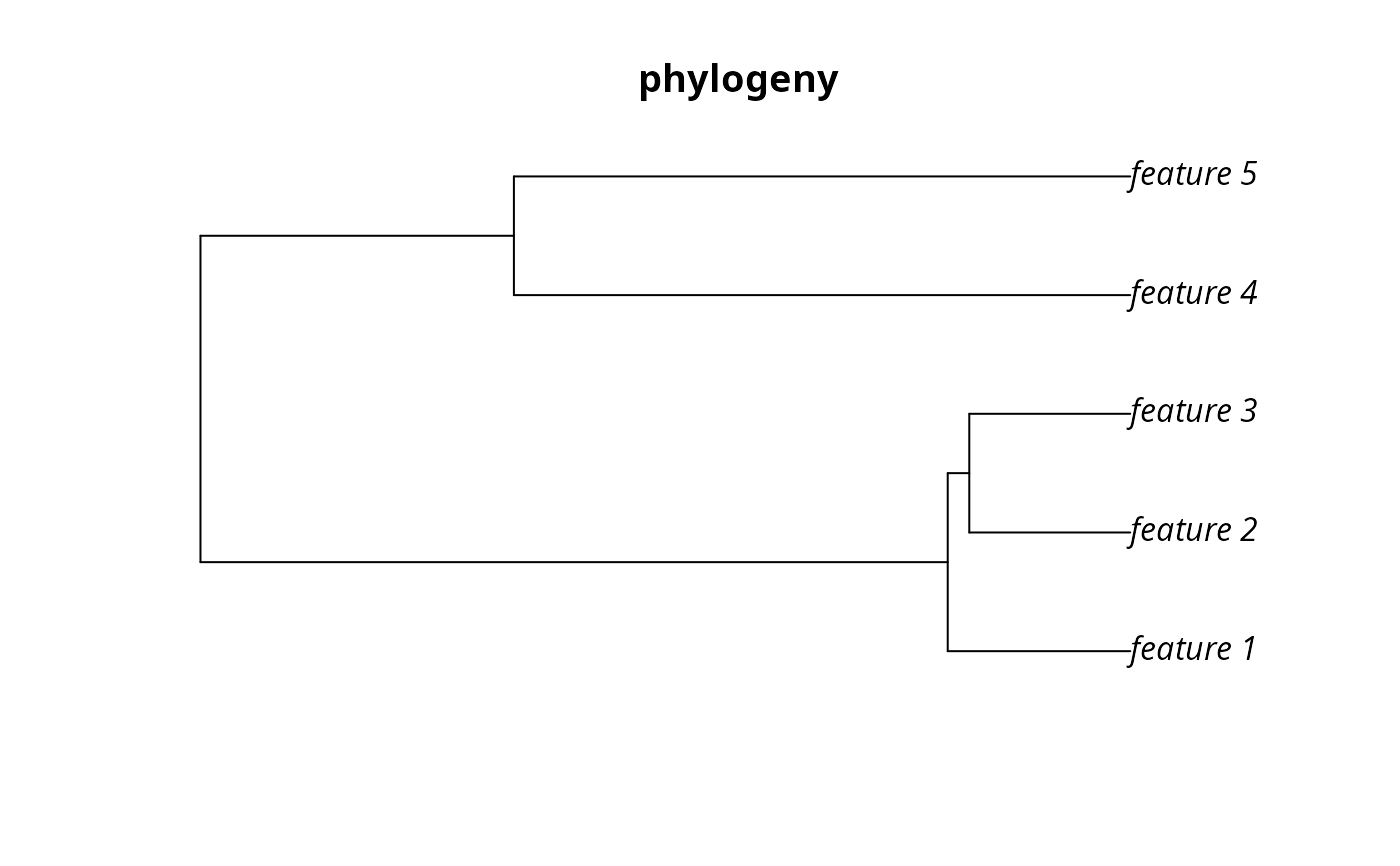# }
# create problem with a maximum phylogenetic endemism objective,
# where each feature needs 10% of its distribution to be secured for
# it to be adequately conserved and a total budget of 1900
p1 <- problem(sim_pu_raster, sim_features) %>%
# \dontrun{
# solve problem
s1 <- solve(p1)

# plot solution
plot(s1, main = "solution", axes = FALSE, box = FALSE)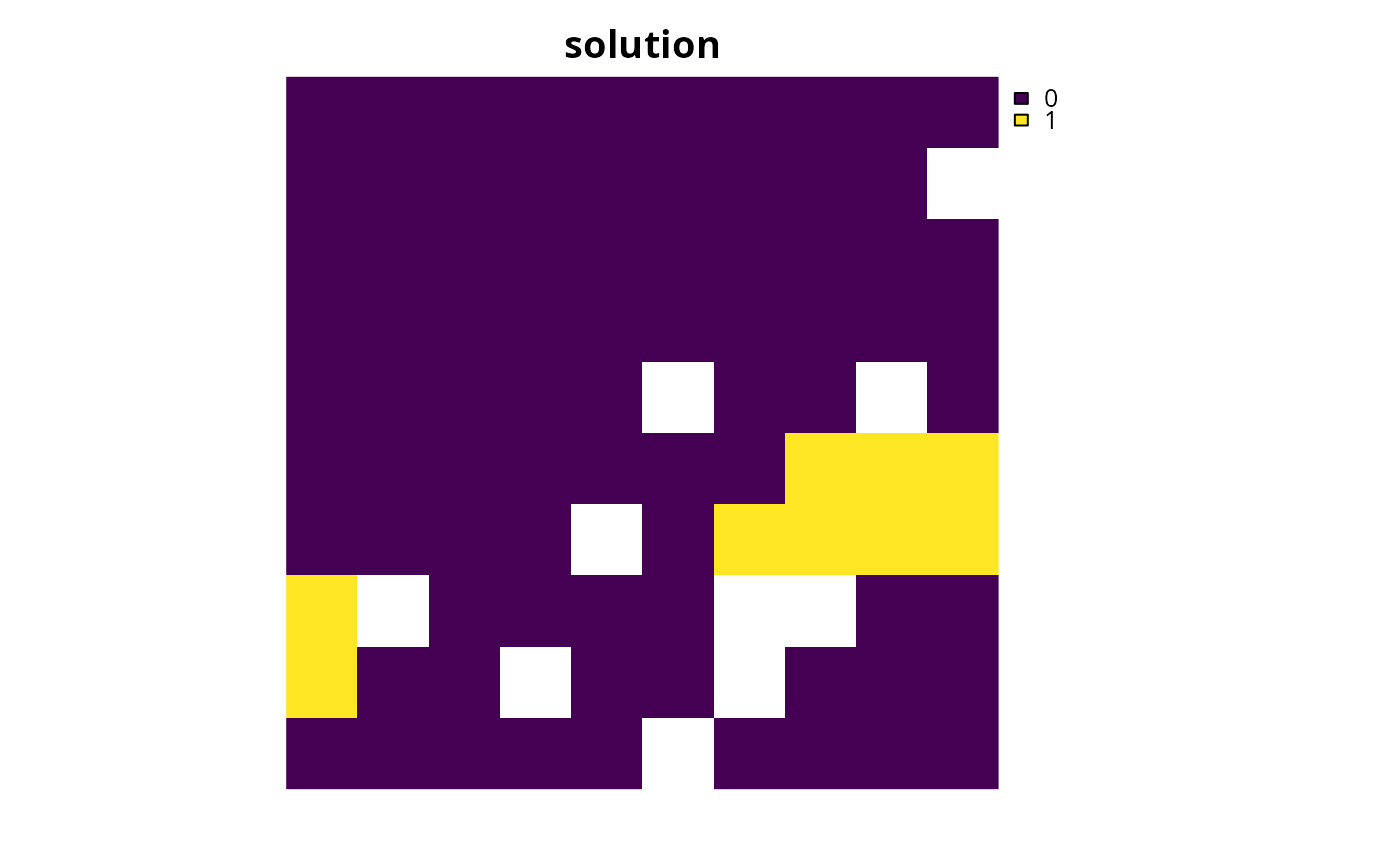# find out which features have their targets met
r1 <- eval_target_coverage_summary(p1, s1)
print(r1, width = Inf)
#> # A tibble: 5 × 9
#>   feature met   total_amount absolute_target absolute_held absolute_shortfall
#>   <chr>   <lgl>        <dbl>           <dbl>         <dbl>              <dbl>
#> 1 layer.1 FALSE         83.3            8.33          7.71              0.618
#> 2 layer.2 FALSE         31.2            3.12          2.35              0.775
#> 3 layer.3 FALSE         72.0            7.20          5.81              1.38
#> 4 layer.4 TRUE          42.7            4.27          4.28              0
#> 5 layer.5 TRUE          56.7            5.67          5.77              0
#>   relative_target relative_held relative_shortfall
#>             <dbl>         <dbl>              <dbl>
#> 1             0.1        0.0926            0.00742
#> 2             0.1        0.0752            0.0248
#> 3             0.1        0.0808            0.0192
#> 4             0.1        0.100             0
#> 5             0.1        0.102             0

# plot the phylogeny and color the adequately represented features in red
plot(sim_phylogeny, main = "adequately represented features",
tip.color = replace(
rep("black", nlayers(sim_features)),
sim_phylogeny$tip.label %in% r1$feature[r1$met], "red"))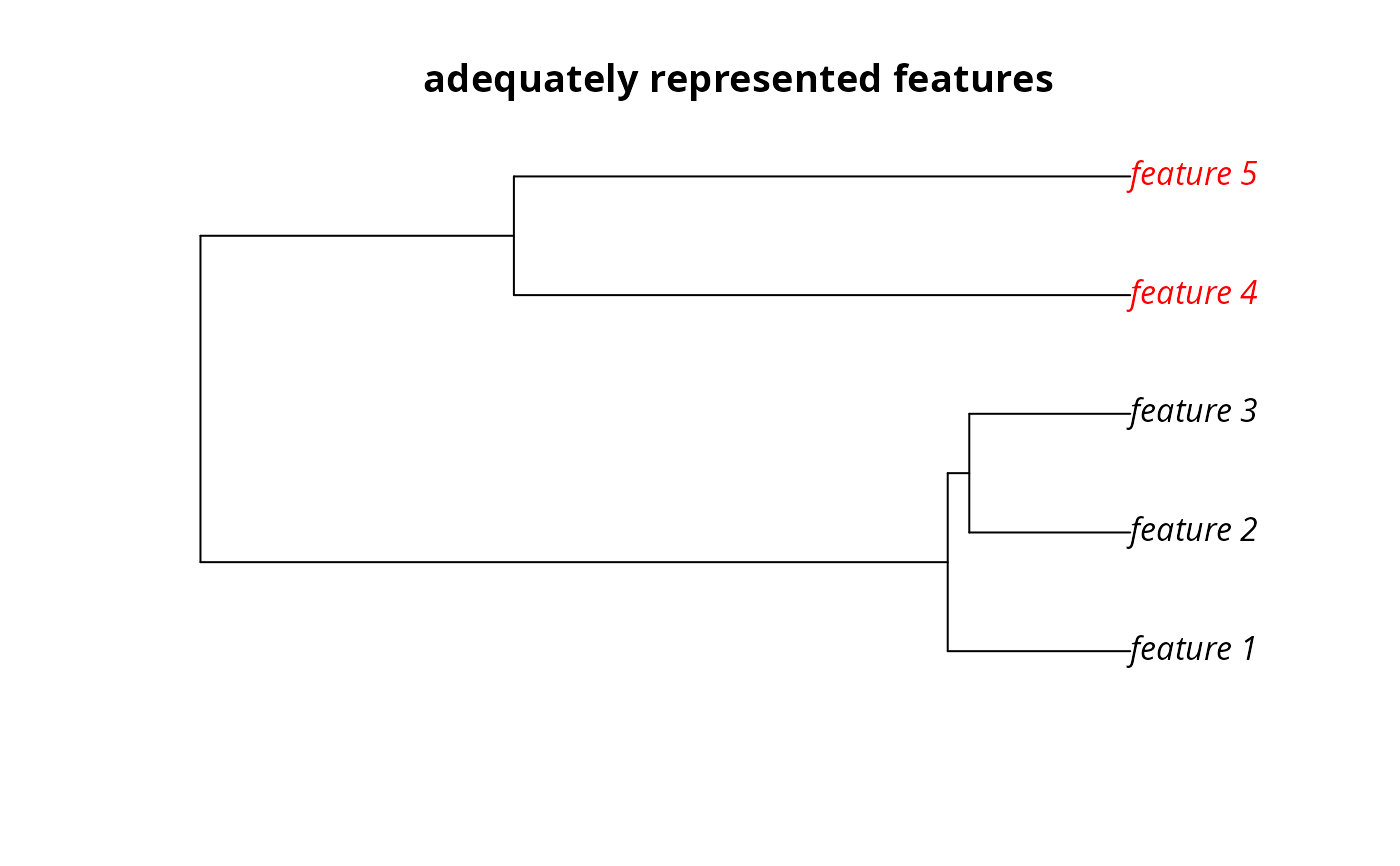# } # rename the features in the example phylogeny for use with the # multi-zone data sim_phylogeny$tip.label <- feature_names(sim_features_zones)

# create targets for a multi-zone problem. Here, each feature needs a total
# of 10 units of habitat to be conserved among the three zones to be
targets <- tibble::tibble(
feature = feature_names(sim_features_zones),
zone = list(zone_names(sim_features_zones))[rep(1,
number_of_features(sim_features_zones))],
type = rep("absolute", number_of_features(sim_features_zones)),
target = rep(10, number_of_features(sim_features_zones)))

# create a multi-zone problem with a maximum phylogenetic endemism
# objective, where the total expenditure in all zones is 5000.
p2 <- problem(sim_pu_zones_stack, sim_features_zones) %>%
# \dontrun{
# solve problem
s2 <- solve(p2)

# plot solution
plot(category_layer(s2), main = "solution", axes = FALSE, box = FALSE)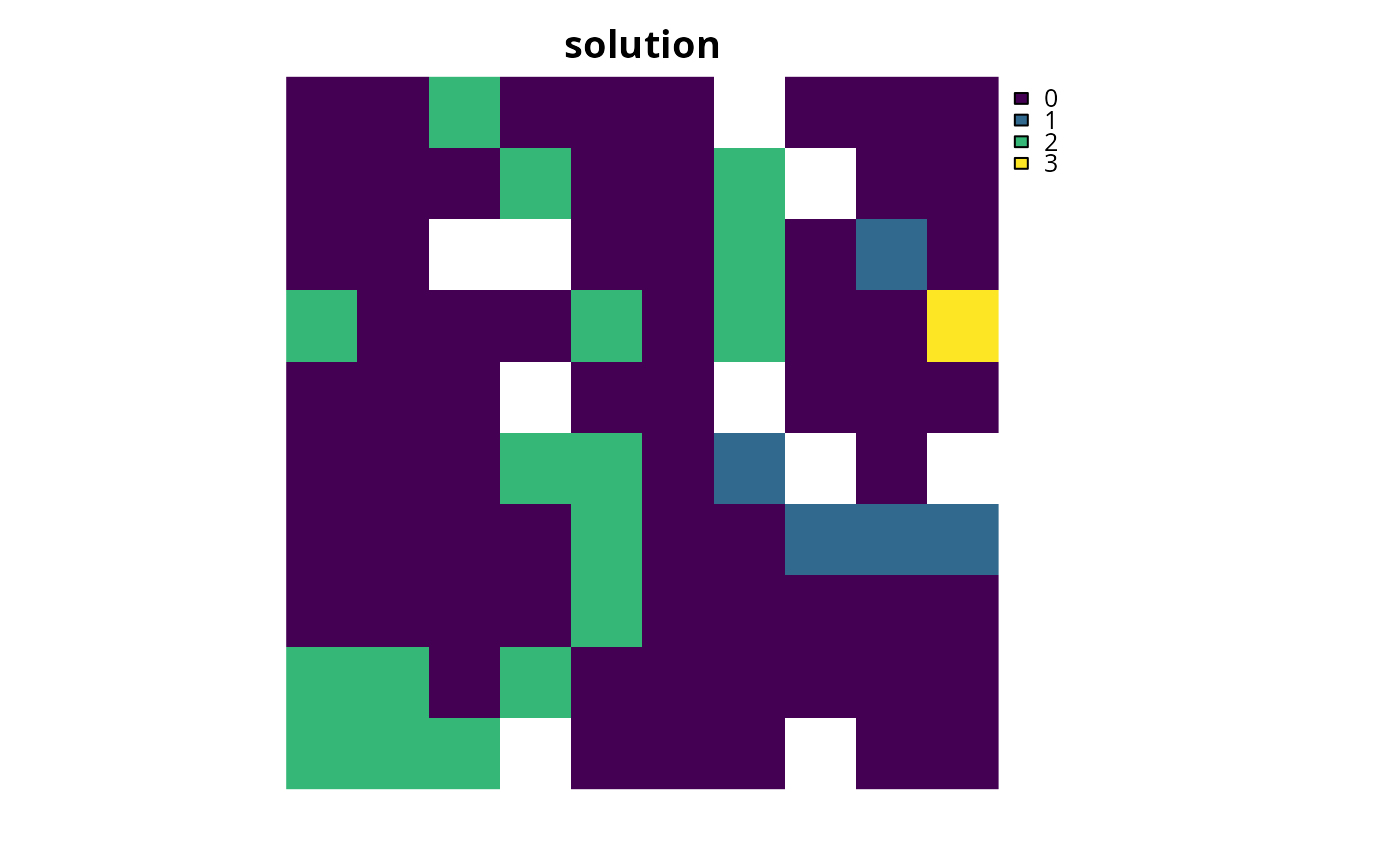# find out which features have their targets met
r2 <- eval_target_coverage_summary(p2, s2)
print(r2, width = Inf)
#> # A tibble: 5 × 11
#>   feature   zone      sense met   total_amount absolute_target absolute_held
#>   <chr>     <list>    <chr> <lgl>        <dbl>           <dbl>         <dbl>
#> 1 feature_1 <chr > >=    TRUE         250.               10         19.6
#> 2 feature_2 <chr > >=    FALSE         93.6              10          6.72
#> 3 feature_3 <chr > >=    TRUE         216.               10         16.3
#> 4 feature_4 <chr > >=    TRUE         128.               10         10.0
#> 5 feature_5 <chr > >=    TRUE         170.               10         13.7
#>   absolute_shortfall relative_target relative_held relative_shortfall
#>                <dbl>           <dbl>         <dbl>              <dbl>
#> 1               0             0.0400        0.0786             0
#> 2               3.28          0.107         0.0717             0.0351
#> 3               0             0.0463        0.0753             0
#> 4               0             0.0781        0.0782             0
#> 5               0             0.0588        0.0807             0

# plot the phylogeny and color the adequately represented features in red
plot(sim_phylogeny, main = "adequately represented features",
tip.color = replace(rep("black", nlayers(sim_features)),
which(r2$met), "red"))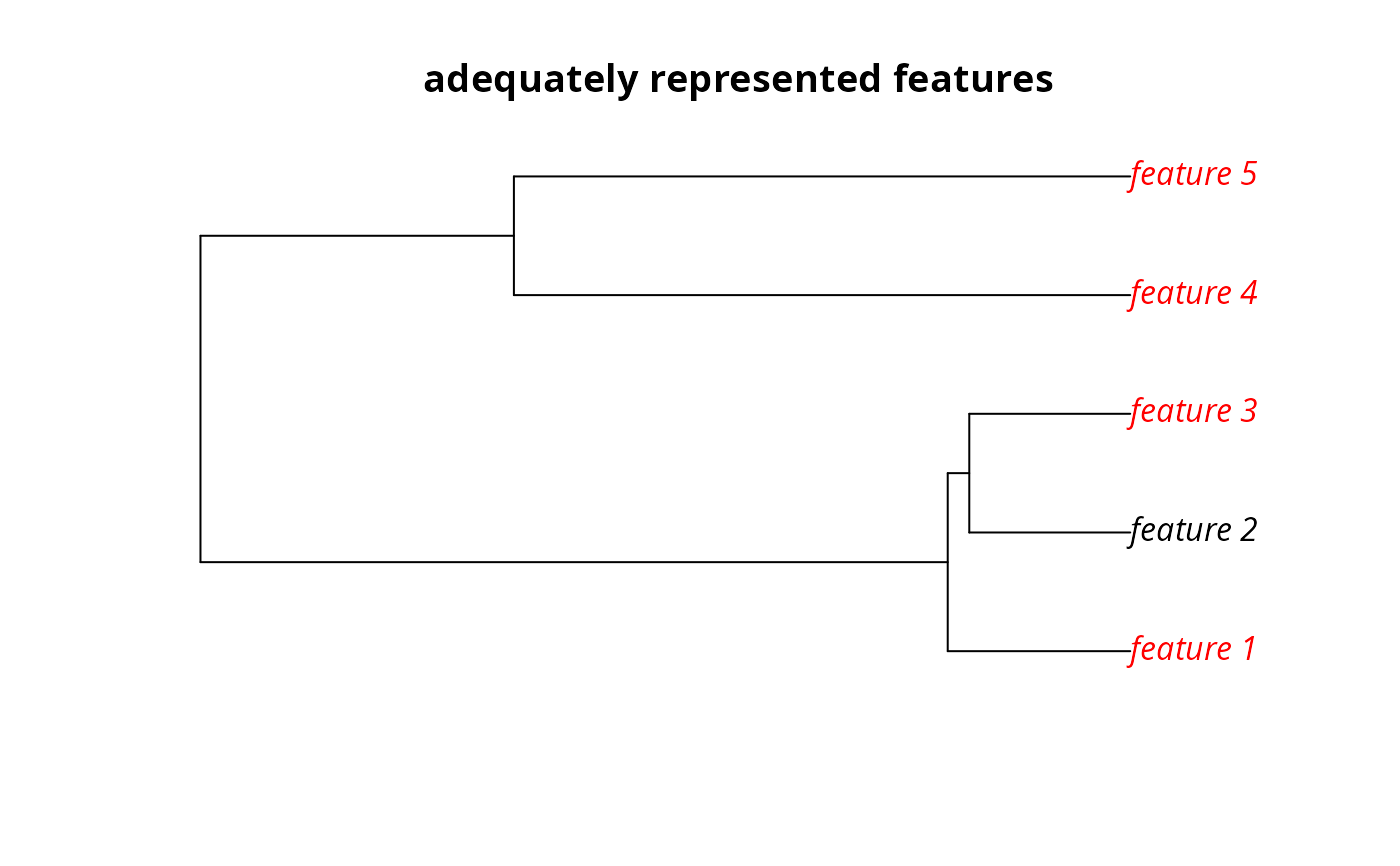# } # create a multi-zone problem with a maximum phylogenetic endemism # objective, where each zone has a separate budget. p3 <- problem(sim_pu_zones_stack, sim_features_zones) %>% add_max_phylo_end_objective(c(2500, 500, 2000), sim_phylogeny) %>% add_manual_targets(targets) %>% add_binary_decisions() %>% add_default_solver(verbose = FALSE) # \dontrun{ # solve problem s3 <- solve(p3) # plot solution plot(category_layer(s3), main = "solution", axes = FALSE, box = FALSE)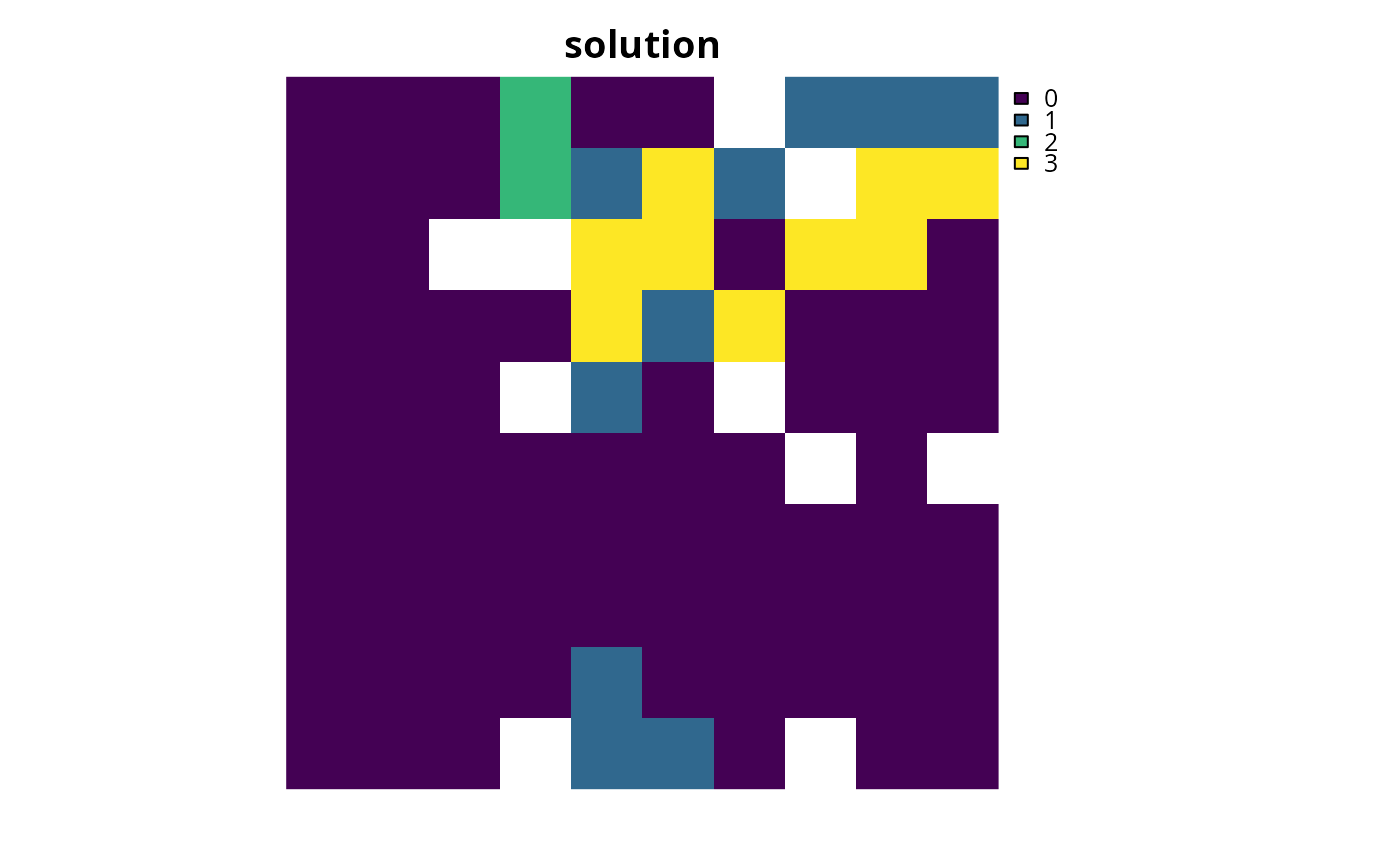# find out which features have their targets met r3 <- eval_target_coverage_summary(p3, s3) print(r3, width = Inf) #> # A tibble: 5 × 11 #> feature zone sense met total_amount absolute_target absolute_held #> <chr> <list> <chr> <lgl> <dbl> <dbl> <dbl> #> 1 feature_1 <chr > >= TRUE 250. 10 15.7 #> 2 feature_2 <chr > >= FALSE 93.6 10 5.73 #> 3 feature_3 <chr > >= TRUE 216. 10 12.1 #> 4 feature_4 <chr > >= TRUE 128. 10 10.0 #> 5 feature_5 <chr > >= TRUE 170. 10 11.4 #> absolute_shortfall relative_target relative_held relative_shortfall #> <dbl> <dbl> <dbl> <dbl> #> 1 0 0.0400 0.0628 0 #> 2 4.27 0.107 0.0612 0.0456 #> 3 0 0.0463 0.0559 0 #> 4 0 0.0781 0.0782 0 #> 5 0 0.0588 0.0669 0 # plot the phylogeny and color the adequately represented features in red plot(sim_phylogeny, main = "adequately represented features", tip.color = replace(rep("black", nlayers(sim_features)), which(r3$met), "red"))# }